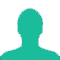# In an examination 80% candidates passed in English and 85% candidates passed in Mathematics. If 73% candidates passed in both these subjects, then what per cent of candidates failed in both the subjects?

A. 8

B. 15

C. 27

D. 35

### Solution(By Examveda Team)

Students passed in English = 80%
Students passed in Math's = 85%
Students passed in both subjects = 73%
Then, number of students passed in at least one subject
= (80+85)-73
= 92%. [The percentage of students passed in English and Maths individually, have already included the percentage of students passed in both subjects. So, We are subtracting percentage of students who have passed in both subjects to find out percentage of students at least passed in one subject.]

Thus, students failed in both subjects = 100-92 = 8%.

This Question Belongs to Arithmetic Ability >> Percentage

1.Total percentage =100
Pass E=80% m=85% both=73%
(E-both subject) 80-73=7
(M-both subject) 85-73= 12
73+12+7=92
92-100=8
=8%

2.Its so simple out of 100% 80% student passed in English and 85% passed in maths.73% passed in both.it mean out of 100% 73% passed in both,7% passed only in English(80-73) and 12% only in math(85-73).so after that left only 8 student who failed (100-73-7-12)

3.that's right

4.E union M,=(E+M)-(E intersection M)
let English represented by E
mathematics by M
put valu
=(80+85)-73
=92
number of students which is pass in both subject 92%
fail =total -pass
=100-92
=8%

5.8%

6.If price of petrol increases by 35% and Rajesh intends to spend only an additional 25% on petrol, by how much % will he reduce the quantity of petrol purchased?(Approx)

7.Use Set theory the problem will be easier

8.Passed in english -80%
failed in english-(100-80)%
-20%
passed in maths -85%
failed in maths -(100-85)%
-15%
Total passed -100%-(20+15)%
-100%-35%
-65%
therefore,failed-73%-65%
-8%
ans.percentage-8

9.passed both only passed
maths 85 73 12
eng 80 73 7
total passed in both >>>12+7+73=92%
stu failed in both >>>> 100%-92%=8%

10.okay if they give no. students instead of each percentage,ans need to be in percentage means how to solve

11.how is it posiable 92%student pass one sub. plese explan it

Related Questions on Percentage